# Point of inflection

A pointon a planar curve having the following properties: atthe curve has a unique tangent, and within a small neighbourhood aroundthe curve lies within one pair of vertical angles formed by the tangent and the normal (Fig. a). normal tangentFigure: p073190a

Let a functionbe defined in a certain neighbourhood around a pointand let it be continuous at that point. The pointis called a point of inflection forif it is simultaneously the end of a range of strict convexity upwards and the end of a range of strict convexity downwards. In that case the point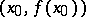is called a point of inflection on the graph of the function, i.e. the graph ofat"inflects" through the tangent to it at that point; for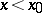the tangent lies under the graph of, while for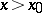it lies above that graph (or vice versa, Fig. b).Figure: p073190b

A necessary existence condition for a point of inflection is: Ifis twice differentiable in some neighbourhood of a point, and ifis a point of inflection, then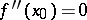. A sufficient existence condition for a point of inflection is: Ifistimes continuously differentiable in a certain neighbourhood of a point, withodd and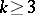, while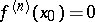for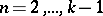, and, thenhas a point of inflection at.